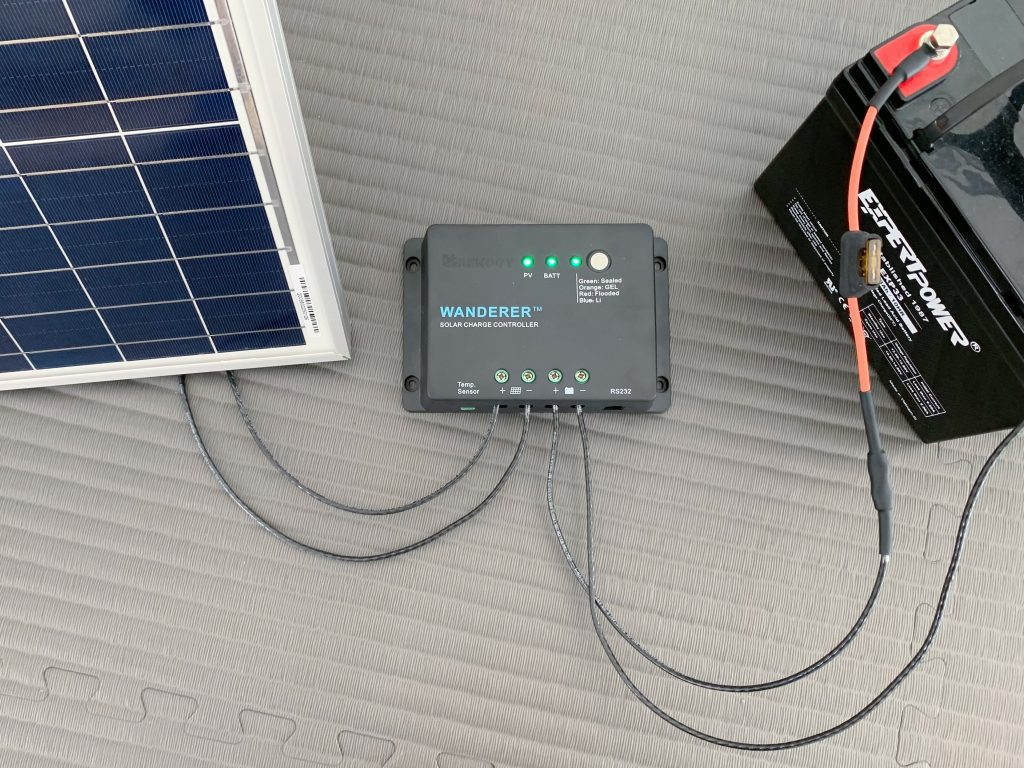# Solar Panel Charge Time Calculator

## Solar Panel Charge Time Calculator

Error: Enter a valid voltage
Error: Enter a valid battery capacity
Error: Select a battery type
Error: Enter a valid wattage
Error: Select a charge controller type
Estimated charge time:
of direct sunlight

## Solar Battery Charging FAQ

### How long does it take to charge a battery with a solar panel?

Use the calculator at the top of the page. It takes into account nearly every factor to give you an estimate for your specific setup.

Let's say you have the following solar power system:

• 12 volt, 30Ah lead acid battery discharged to 50%
• 100 watt solar panel
• PWM charge controller

Here's a simplified way to estimate how long it'd take for the solar panel to charge the battery:

1. Divide solar panel wattage by battery voltage to estimate maximum charge current output by solar charge controller:

`100W / 12V = 8.33A`

2. Multiply current by rule-of-thumb system losses (20%) and charge controller efficiency (PWM: 75%; MPPT: 95%):

`8.33A * (1 - 20%) * 75% = 5A`

3. Multiply battery capacity by 1 divided by rule-of-thumb battery charge efficiency (lead acid: 85%; lithium: 95%):

`30Ah * (1 / 85%) = 35.29Ah`

4. Divide battery capacity by current to estimate how long it'd take to charge the entire battery:

`35.29Ah / 5A = 7.06 hrs`

5. Multiply the charge time by the battery's depth of discharge to estimate how long it'd take to charge the battery at its current level:

`7.06 hrs * 50% DoD = 3.53 hrs`

6. Add 2 hours to account for the absorption charging stage of most charge controllers:

`3.53 hrs + 2 hrs = 5.53 hrs`

So, in this example, it'd take about 5.5 hours to charge a 12 volt battery with a 100 watt solar panel.

Note: Our solar charging calculator, which takes into account more factors, estimates it'd take 6.6 hours for this setup.

### How do you charge a battery with a solar panel?To charge a battery with a solar panel, you connect both the battery and solar panel to a solar charge controller.

Never connect a solar panel directly to a battery. Doing so can damage the battery.

Instead, connect the battery and then solar panel (in that order!) to a solar charge controller. Charge controllers regulate the current and voltage coming from solar panels to safely charge the battery.

Step-by-step instructions: How to Connect a Battery to a Solar Panel

## More Solar Calculators